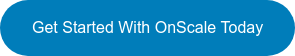# OnScale Blog

Our blog covers tips for using OnScale, new features and developments, and upcoming events and webinars.  Subscribe and get the latest posts in your inbox.

# Practice: How to Calculate Piezoelectric Material Properties from a Material Datasheet

In Part 1 of this Blog Tutorial we provided a more theoretical example of piezoelectric equations and the important coefficients to know.

If you missed Part 1 you can check it out here!

Now let’s start with a practical consideration:

What parameters do we need in OnScale to define a Piezoelectric material?

The accurate modelling of piezoelectric materials requires 13 independent parameters:

If we look at the material properties in OnScale for a material such as pmt3, we will require the following:## How to calculate the stiffness or compliance coefficients?

Let’s consider the isotropic case. Most metallic alloys and thermoset polymers are considered isotropic which means that the material properties are independent of direction.

Such materials have only 2 independent variables (i.e. elastic constants) in their stiffness and compliance matrices, as opposed to the 21 elastic constants in the general anisotropic case.

The two elastic constants are usually expressed as the Young's modulus E and the Poisson's ratio ν. However, the alternative elastic constants K (bulk modulus) and/or G (shear modulus) can also be used. For isotropic materials, G and K can be found from E and n by a set of equations, and vice-versa.

Hooke's law for isotropic materials in compliance matrix form is given by:Some literatures may have a factor 2 multiplying the shear moduli in the compliance matrix resulting from the difference between shear strain and engineering shear strain, where the stiffness matrix is equal to the inverse of the compliance matrix, and is given by:Now… remember, this is all for the isotropic case!

A piezoelectric material is not isotropic so those relations will be slightly more complex.

Now, let’s go back to the example on the datasheet provided in Part 1.

Which parameter do we already have on the material datasheet and which parameter should we calculate?

For this material datasheet, we see that we have the density, the mechanical Q and the 2 dielectric constants (also called relative permittivity).

We will need to calculate the stiffness matrix (1) using the young's modulus and Poisson's ratio provided. Then, we will need to calculate the matrix [e] (2) of piezoelectric coefficients from the matrix [d] provided.### 1- Calculation of the stiffness matrix

First, we need to calculate the compliance matrix and then we can inverse it to obtain the stiffness matrix. As PZT materials exhibits transverse isotropy (6mm crystal symmetry), we can use the following matrix to calculate the compliance matrix:The transverse modulus G12 is related to the transverse Poisson's ratio and the transverse stiffness through the following equation:Here is a python script that you can use to calculate this compliance matrix and the associated stiffness matrix (which is the inverse of the compliance matrix).Note: To run this script, you will need to install Python first and then the module numpy of python.

This is what you should obtain:

Compliance MatrixStiffness Matrix

###2- Calculation of Piezoelectric [e] coefficients from [d]

Now that we have the Stiffness Matrix, we can calculate the coefficient [e] from the coefficient [d] written on the material datasheet.To transform those [d] coefficients, we need to have a look at some of the transformation relations mentioned on this page from the efunda website:

Stress-Charge to Strain-Charge:This tells us the following:

• To calculate [e], we need to use the relation [e] = [d].[c]
• [c] is the stiffness matrix calculated previously

Note: [c] is the stiffness matrix, NOT the compliance matrix, it's a bit confusing

We can now use the following Python script to calculate:Which provides us with the following [e] coefficientsNow that we have all the coefficients, we are ready to input this new material in OnScale

### How to create a new Piezoelectric material in OnScale?

To add this new piezoelectric material into the global onscale database, follow the steps below in the material database:

1. Click on Add Global Material
2. Add a Material Description
3. Add a Material Name (It must be unique)
4. Choose a Material Category and a Material Model
5. Double click on the coefficients you want to modify
6. Click on SaveWe hope from this you will now be able to Calculate Piezoelectric Material Properties from Material Datasheet. If you have any questions let us know in the comment section below!###### Cyprien Rusu, Director of Engineering for Asia at OnScale
Cyprien Rusu is our Director of Engineering for Asia at OnScale. He has a extensive background in FEM, Technical Marketing, Sales and Support. Cyprien recieved his MS in Civil Engineering from Tsinghua University. At OnScale he is a trusted advisor for our client base in Asia, while creating and providing OnScale training.